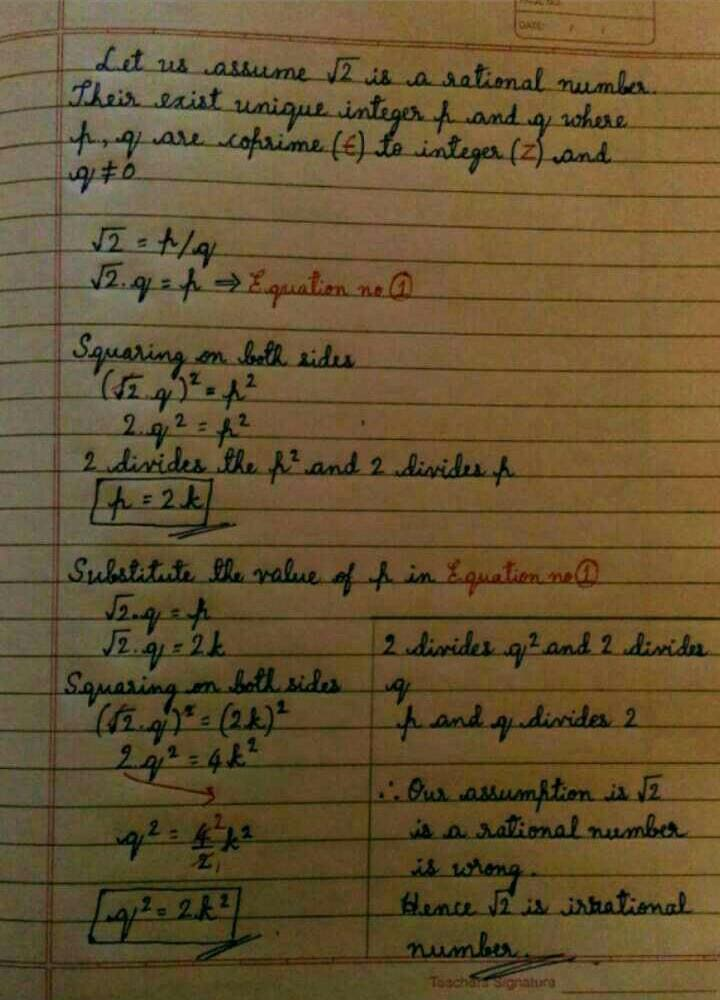Courses

# Prove that root 2 is irrational?

## Class 10 Question☙ᴍʀ sᴛʀᴀɴɢᴇʀ☙ Feb 24, 2021
The proof that √2 is indeed irrational is usually found in college level math texts, but it isn't that difficult to follow. It does not rely on computers at all, but instead is a "proof by contradiction": if √2 WERE a rational number, we'd get a contradiction.Kuldeep Raj 3 weeks agoLighτ Yαmɪ Mar 25, 2021
Let us assume √2 is rational number hence it can be written in the form
√2=p/q where p and q are co prime and q≠0
squaring both side
2=p²/q²
p²=2q²
here 2 is a factor of p² hence it is also a factor of p
putting p=2a
4a²=2q²
q²=2a²
here 2 is also a factor of q
but p and q are co prime hence it contradicts our assumption that p and q are co prime and √2 is rational
hence, we can say that √2 is irrational

This discussion on Prove that root 2 is irrational? is done on EduRev Study Group by Class 10 Students. The Questions and Answers of Prove that root 2 is irrational? are solved by group of students and teacher of Class 10, which is also the largest student community of Class 10. If the answer is not available please wait for a while and a community member will probably answer this soon. You can study other questions, MCQs, videos and tests for Class 10 on EduRev and even discuss your questions like Prove that root 2 is irrational? over here on EduRev! Apart from being the largest Class 10 community, EduRev has the largest solved Question bank for Class 10.